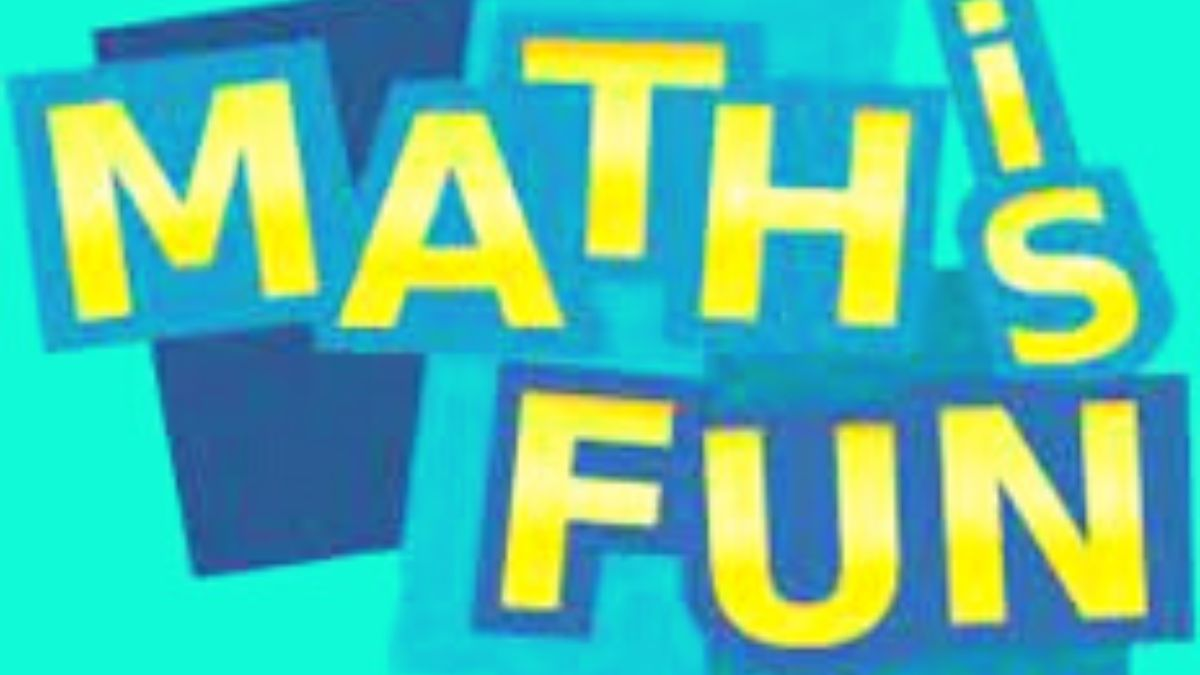# Math riddles with answers: Math is an incredibly tough subject, but it can be incredibly fun too! These math riddles are a proof!

We have already talked about why math is not liked by many, let's talk about what makes math a fun subject. These fun math riddles are all what you need today!Math is fun. Try these math riddles!

Math is often seen as a tough subject. However, it can be fun too.

Ever wondered why some people excel at math, while others simply hate it? The present-day education system tries hard to make learning fun and digestible. While the syllabus has become even more comprehensive, schools and colleges have now incorporates smart methods to teach complex concepts. Have a look at the books of children of this era, or attend any of their classes, and you will feel that technology is really helping kids with learning. If the very same learning culture had been there back in the time when you were a student, many of your class students who struggled with math could not only score well but also enjoy the subject more. Sadly, math has always been seen as a subject that is incredibly tough but not as a subject that is full of possibilities.

These Math Riddles, however, are fun and engaging and might alter the ways you look at the subject.

If 1= 3

2 = 3

3= 5

4 = 4

5= 4

7= 5

Then what is 6?

## Math Riddle 2:

How many feet are there in a mile?

Math Riddle 1:
If 1= 3

2 = 3

3= 5

4 = 4

5= 4

7= 5

Then what is 6?

6 = 3.

This is because the number has 3 digits.

Math Riddle 2:

How many feet are there in a mile